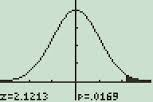# Robust Statistics / Estimation (Robustness) & Breakdown Point

Share on

Statistics Definitions > Robust Statistics

## What are Robust Statistics?

Robust statistics are resistant to outliers. In other words, if your data set contains very high or very low values, then some statistics will be good estimators for population parameters, and some statistics will be poor estimators. For example, the mean is very susceptible to outliers (it’s non-robust), while the median is not affected by outliers (it’s robust).

## Robust & Non-Robust Estimators

(Click on a term for the main article)
Robust Estimators:

Non-robust Estimators:

Robust Statistics are different from robust tests, which are defined as tests that will still work well even if one or more assumptions are altered or violated. For example, Levene’s test for equality of variances is still robust even if the assumption of normality is violated.

## When You Shouldn’t Rely on Robustness.Robust statistics work on the assumption that your data follows a normal distribution.

Robust statistics assume that your underlying distribution is normal, so you shouldn’t use them for skewed or multimodal distributions. These statistics work on the assumption that the underlying data is approximately normal; if you use these statistics on a differently-shaped distribution, they will give misleading results. That said, they don’t work well for all normally shaped distributions, like mixtures of two normal distributions (called a contaminated distribution).

While robust statistics are resistant to outliers, they are not always appropriate for the same reason; it also means that the statistics you present give no idea about outliers. For example, the median house price where I live is about \$250,000. That doesn’t sound too impressive, and you could be forgiven for thinking I must live in a pretty “average” town. However, I live by the river, and while most homes sell for about that price, about 1% of homes are on the river and sell for \$2-3 million.

## Breakdown Point

A breakdown point is the point after which an estimator becomes useless. It is a measure of robustness; The larger the breakdown point, the better the estimator. If an estimator has a high breakdown point, it may be called a resistant statistic.

There are two types of breakdown points: finite sample breakdown points and asymptotic breakdown points.

## Finite Sample Breakdown Points

The finite sample breakdown point is defined as the fraction of data which can be given arbitrary values without making the estimator, arbitrarily too large or too small. It is usually dependent on the sample size, n, and can be written as a function of n.

As an example, consider the arithmetic mean as the estimator of a data set. It is given by ( x1 + x2 + … + xn )/n. You can change the calculated value of the mean by an arbitrarily large amount, simply by changing one of the data points by a large amount. Therefore, the breakdown point is just 1/n.

## Asymptotic Breakdown Points

The asymptotic breakdown point is what is usually referred to when the term ‘breakdown point’ is used, and it is the finite sample breakdown point as n goes to infinity.

In the example above, 1/n approaches 0 as n approaches infinity, so the (asymptotic) breakdown point of the mean is just 0. This tells us that the mean, as an estimate, is not at all robust or resistant. This is quite the opposite of the median, which has the highest possible breakdown point, of 1/2 (Wilcox, 2010).

## References

Geyer, Charles. Break down Point Theory Notes. Retrieved from http://www.stat.umn.edu/geyer/5601/notes/break.pdf on June 23, 2018

Davies & Gather. The Breakdown Point– Examples and Counterexamples. REVSTAT – Statistical Journal, Volume 5, Number 1, March 2007, 1–17. Retrieved from https://www.ine.pt/revstat/pdf/rs070101.pdf on June 23, 2018

Sakata & White. Breakdown Point. Encyclopedia of Statistical Sciences. First published: 15 August 2006 https://doi.org/10.1002/0471667196.ess0607.pub2. Retrieved from https://onlinelibrary.wiley.com/doi/full/10.1002/0471667196.ess0607.pub2 on June 23, 2018.

Wilcox, R. (2010). Fundamentals of Modern Statistical Methods: Substantially Improving Power and Accuracy. Springer Science and Business Media.

CITE THIS AS:
Stephanie Glen. "Robust Statistics / Estimation (Robustness) & Breakdown Point" From StatisticsHowTo.com: Elementary Statistics for the rest of us! https://www.statisticshowto.com/robust-statistics/
---------------------------------------------------------------------------Need help with a homework or test question? With Chegg Study, you can get step-by-step solutions to your questions from an expert in the field. Your first 30 minutes with a Chegg tutor is free!